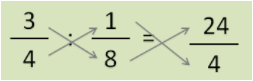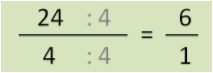Smartick is an online platform for children to master math in only 15 minutes a day

Oct19

# Word Problems with Division of Fractions

Today we are going to look at problems involving the division of fractions.

Are you ready to begin? Well, the first thing that we must remember is that in a problem with fractions we should follow all the necessary steps to solve any problem, only we have to add a step for the simplification of the result:

3. Think about the details we need.
4. Carry out the operation. In this case, you should know how to divide fractions
5. Simplify the results, if possible.
6. Think about whether the result makes sense.

Now that we have already recalled what is fundamental for solving a problem with fractions, we can move on to review word problems with division of fractions. We will look at a problem where it is necessary to divide fractions to solve.

Let’s look at an example:

Priscilla bought cheese that weighs ¾ pounds. If she divides it into portions that are each 1/8 pound, how many portions can she make?

The first thing we should do are steps 1, 2, and 3: read carefully, understand the question, and think about the relevant details.

Priscilla bought cheese that weighs ¾ of a pound. If she divides it into portions that are each 1/8 of a pound, how many portions can she make?

That is to say…

If she divided ¾ of a pound into equal portions of 1/8 of a pound, how many portions did she make?

We already know that the operation is division. We are going to move on to steps 4 and 5 (solve and simplify).

If she divided ¾ of a pound into equal portions of 1/8 of a pound, how many portions did she make?

We divide by taking the reciprocal of the second fraction and multiplying:Then we simplify: